Next: Worked example 11.4: Energy Up: Oscillatory motion Previous: Worked example 11.2: Block

## Worked example 11.3: Block and two springs

Question: A block of mass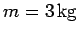is attached to two springs, as shown below, and slides over a horizontal frictionless surface. Given that the force constants of the two springs are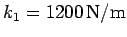and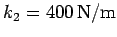, find the period of oscillation of the system.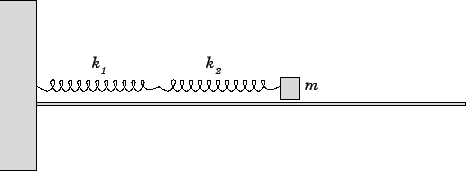Answer: Let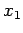and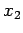represent the extensions of the first and second springs, respectively. The net displacementof the mass from its equilibrium position is then given by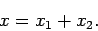Let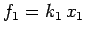and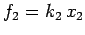be the magnitudes of the forces exerted by the first and second springs, respectively. Since the springs (presumably) possess negligible inertia, they must exert equal and opposite forces on one another. This implies that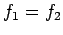, or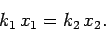Finally, ifis the magnitude of the restoring force acting on the mass, then force balance implies that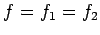, or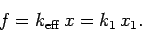Here,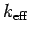is the effective force constant of the two springs. The above equations can be combined to give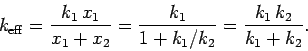Thus, the problem reduces to that of a block of massattached to a spring of effective force constant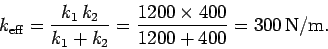The angular frequency of oscillation is immediately given by the standard formula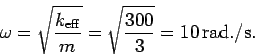Hence, the period of oscillation is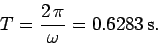Next: Worked example 11.4: Energy Up: Oscillatory motion Previous: Worked example 11.2: Block
Richard Fitzpatrick 2006-02-02